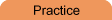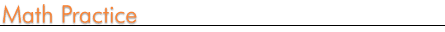Home    |    Teacher    |    Parents    |    Glossary    |    About UsEmail this page to a friendSelect Subject-Select Subject Basic Math Everyday Math Pre-Algebra Algebra Geometry Trigonometry Statistics Calculus Advanced Topics OthersResources· Cool Tools · Formulas & Tables · References · Test Preparation · Study Tips · Wonders of MathSearchHot SubjectsFractionsDecimalsIntegersExponentsInequalitiesPercentsSquare Roots

 Practice Subjects · Basic Math · Everyday Math · Pre-Algebra · Geometry · Algebra · Trigonometry · Statistics · Calculus · Advanced Topics · OthersAlgebra Practice Free! Algebra Worksheet Generator - Generate your own algebra worksheets to print and use. Includes many options and types of equations, systems, and quadratics. Answer sheet included!

 Basic Arithmetic Practice How many can you do? Timed addition, subtraction, multiplication, and division problems for basic math practice.